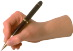the future tense – will

Directions: Write information questions in the future tense using the modal verb "will." First, read the answer. Then, write the question by hand in your notebook.

 Example:Question: What time will the class end?Answer: The class will end at 8:45. 1. Question: What ___________________________? Answer: The movie will end at 1:20. 2. Question: Where __________________________?  Answer: We’ll look for jobs in hotels. 3. Question: How ____________________________? Answer: There will be eight people at the party. 4. Question: How ____________________________? Answer: It will cost \$250 to get my car fixed. 5. Question: When ___________________________? Answer: I’ll call her tomorrow. 6. Question: How ____________________________? Answer: I don’t know how long it will last. 7. Question: When ___________________________? Answer: She’ll graduate in the spring. 8. Question: What ___________________________? Answer: They’ll be here at seven o’clock. 9. Question: Where __________________________? Answer: I’ll be at the grocery store tomorrow. 10. Question: When __________________________? Answer: They won’t finish until after five o’clock.